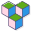CppBuzz# Benison Technologies C & C++ Technical Interview Questions

Question 1:
Write a C++ program to create integer vector of capacity 10 and initialize from 1-10 sequentially

Questions 2:
Write a C++ program to sort the below vector in descending order

``````
vector a = {1.45, 45.1, 54.71, 71.54, 76.12, 12.76};
``````

Question 3:
Define Macro (BITS_SET) to find out 1st and 3rd bit of a byte is set or not? Here is code snippet that will use this macro,

``````
if((BITS_SET(x))
{
printf("Bot bit 1 & 3 are set in byte x");
}
else
{
printf("Either one or none of the bits set in byte x");
}
``````

Question 4:
Is the below code has any issue? if no, what is the output result. If yes, share the fixed code,

``````
char *names;
char n;
int len,i;

for(i=0;i<=2;i++)
{
printf("\n Enter name ");
scanf("%s", n);
len = strlen(n);
*names[i] = malloc(len);
strcpy(names[i], n);
}

for(i=0; i<=5; i++)
printf("\n%s", names[i]);
``````

Question 5:
What is the output of below program?

``````
float a,b,c;
a = b = 3.5;
int x,y,z;
x=y=7;
c = a-b;
printf("result: %d", z = x*y/c);
``````